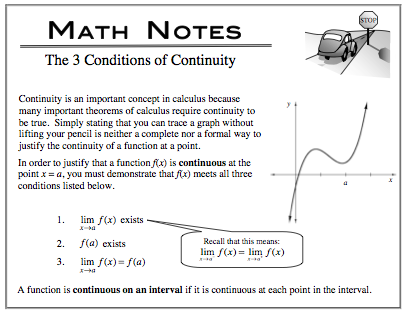### Home > CALC > Chapter 6 > Lesson 6.3.2 > Problem6-95

6-95.

Given the piecewise function $f ( x ) = \left\{ \begin{array} { l l } { \sqrt { 1 - x } + 7 } & { \text { for } x > - 3 } \\ { - a x ^ { 3 } } & { \text { for } x \leq - 3 } \end{array} \right.$, what value of a would make this function continuous?$\sqrt{1-(-3)}+7=-a(-3)^{3}$
Solve for a.

Use the eTool below to visualize the problem.
Click the link at right for the full version of the eTool: Calc 6-95 HW eTool